Pressure Drop

Pressure drop. Laminar flow, horizontal pipe

 www.LMNOeng.com Flow Rate, Q (m3/s): Pipe Inside Diameter, D (m): Pipe Length, L (m): Density, ρ (kg/m3): Dynamic Viscosity, μ (N-s/m2): Pressure Drop, P1-P2 (N/m2): Velocity, V(m/s): Reynolds Number, Re:

Pressure Drop Drawing and Equation: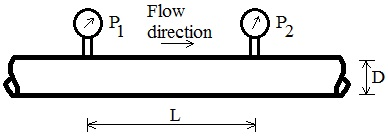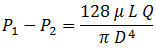Pressure Drop Equation Derivation

For steady flow of an incompressible fluid in a constant diameter horizontal pipe using the Darcy-Weisbach friction loss equation, the energy equation from location 1 to 2 is expressed in terms of pressure drop as: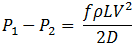where: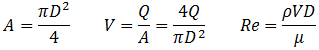When Re < 2100, flow is laminar and: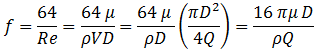Then pressure drop is: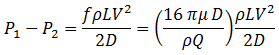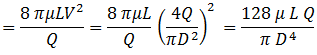The final pressure drop equation is often called Poiseuille's law after the original researcher (Munson et al., 1998, p. 468).

Unit abbreviations, symbols: kg=kilogram, m=meter, N=Newton, s=second. A=Pipe area, f=Moody friction factor.

Reference for pressure drop: Munson, B. R. Young, D. F. and Okiishi, T. H. 1998. Fundamentals of Fluid Mechanics. John Wiley and Sons. Inc. 3ed.